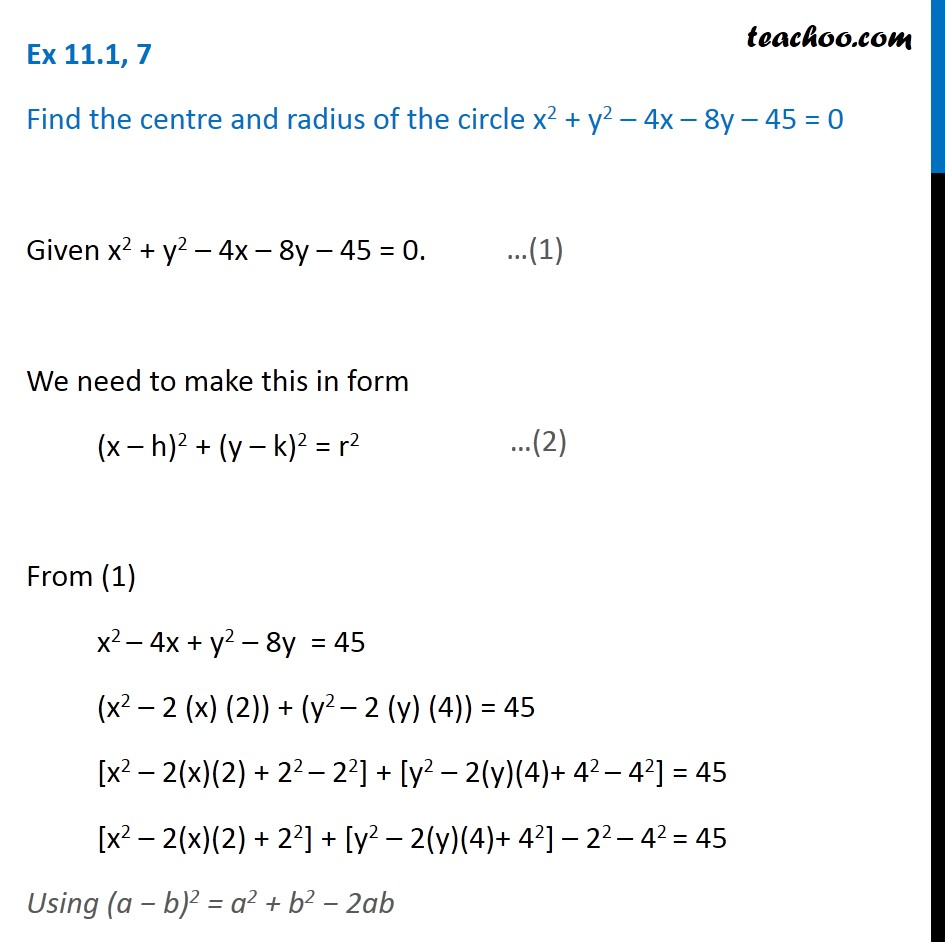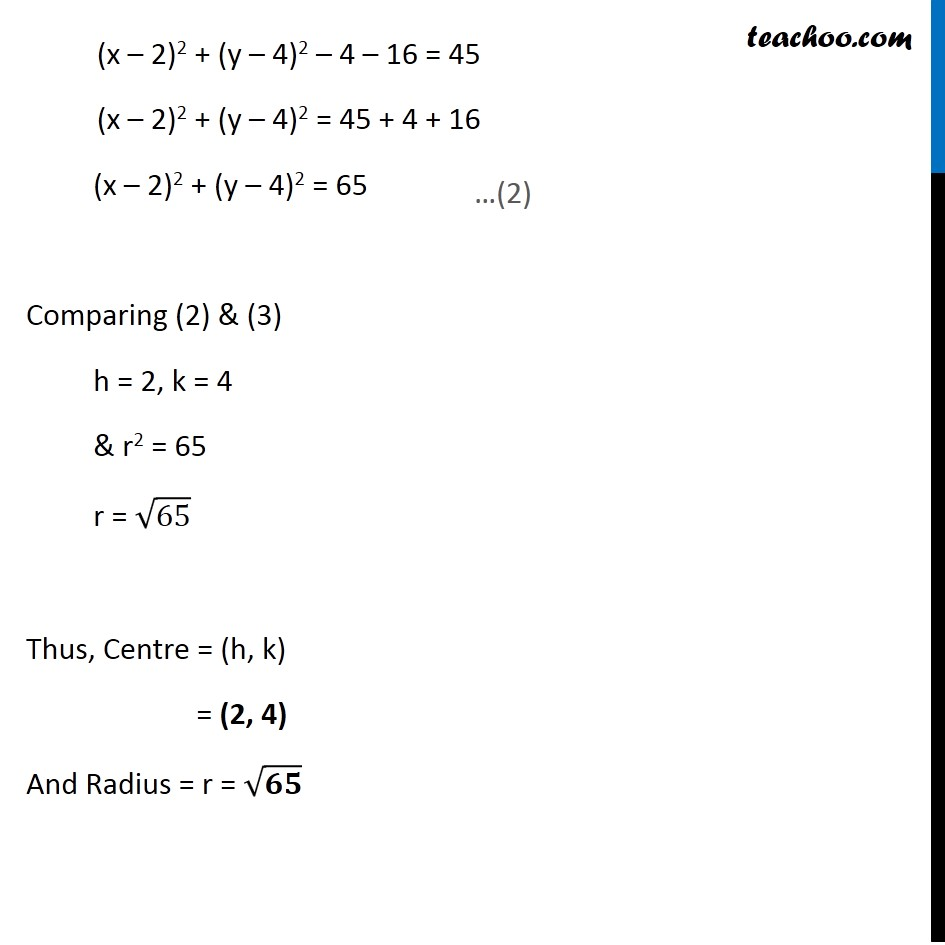Ex 11.1

Chapter 11 Class 11 Conic Sections
Serial order wiseGet live Maths 1-on-1 Classs - Class 6 to 12

### Transcript

Ex 11.1, 7 Find the centre and radius of the circle x2 + y2 – 4x – 8y – 45 = 0 Given x2 + y2 – 4x – 8y – 45 = 0. We need to make this in form (x – h)2 + (y – k)2 = r2 From (1) x2 – 4x + y2 – 8y = 45 (x2 – 2 (x) (2)) + (y2 – 2 (y) (4)) = 45 [x2 – 2(x)(2) + 22 – 22] + [y2 – 2(y)(4)+ 42 – 42] = 45 [x2 – 2(x)(2) + 22] + [y2 – 2(y)(4)+ 42] – 22 – 42 = 45 Using (a − b)2 = a2 + b2 − 2ab (x – 2)2 + (y – 4)2 – 4 – 16 = 45 (x – 2)2 + (y – 4)2 = 45 + 4 + 16 (x – 2)2 + (y – 4)2 = 65 Comparing (2) & (3) h = 2, k = 4 & r2 = 65 r = √65 Thus, Centre = (h, k) = (2, 4) And Radius = r = √𝟔𝟓